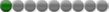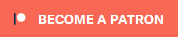# longpinkytoes

Members

39

• #### Last visited

• Rank
Hearthfriend• Gender
Not Telling

## Recent Profile Visitors

697 profile views

• ### Kisuke

1. ...in skyrim, if you have 99% in all skills, what level are you? [the old level cap before legendary was level 81 with 100 in all skills] or how many levels do you get raising all 16 skills from 99% to 100%? per skill: Skill Improve Mult * skill level1.95 + Skill Improve Offset where 991.95 = 7789, nominally (≈) Magic Alchemy - 1.6 * 7,789 + 65 = 12,527 Illusion - 2 * 7,789 + 0 = 15,578 Conjuration - 2 * 7,789 + 0 = 15,578 Destruction - 2 * 7,789 + 0 = 15,578 Restoration - 2 * 7,789 + 0 = 15,578 Alteration - 2 * 7,789 + 0 = 15,578 Combat Enchanting - 1 * 7,789 + 170 = 7,959 Smithing - .25 * 7,789 + 300 = 2,247 Heavy Armor - 2 * 7,789 + 0 = 15,578 Block - 2 * 7,789 + 0 = 15,578 Two-handed - 2 * 7,789 + 0 = 15,578 One-handed - 2 * 7,789 + 0 = 15,578 Stealth Archery - 2 * 7,789 + 0 = 15,578 Light Armor - 2 * 7,789 + 0 = 15,578 Sneak - .5 * 7,789 + 120 = 4,014 Lockpicking - .25 * 7,789 + 300 = 2,247 Pickpocket - .25 * 7,789 + 250 = 2,197 Speech - 2 * 7,789 + 0 = 15,578 tl;dr: 12x - 2 * 7789 + 0 = 186,936 1x - 1.6 * 7789 + 65 = 12,527 1x - 1 * 7789 + 170 = 7,959 1x - .5 * 7,789 + 120 = 4,014 2x - .25 * 7789 + 300 = 2,247 1x - .25 * 7789 + 250 = 2,197 = 215,880xp so getting the last percent in all of your skills took 215,880xp but how many times did you level up earning that much xp? XP required to level up your character = (Current level + 3) * 25 so the amount of experience needed to go from: level 80 to 81 is 83 * 25 = 2075, level 79 to 80 is 82 * 25 = 2050, level 78 to 79 is 81 * 25 = 2025 level 77 to 78 is 80 * 25 = 2000, level 76 to 77 is 79 * 25 = 1975, level 75 to 76 is 78 * 25 = 1950 level 74 to 75 is 77 * 25 = 1925, level 73 to 74 is 76 * 25 = 1900, level 72 to 73 is 75 * 25 = 1875 level 71 to 72 is 74 * 25 = 1850, level 70 to 71 is 73 * 25 = 1825, level 69 to 70 is 72 * 25 = 1800 level 68 to 69 is 71 * 25 = 1775, level 67 to 68 is 70 * 25 = 1750, level 66 to 67 is 69 * 25 = 1725 level 65 to 66 is 68 * 25 = 1700, level 64 to 65 is 67 * 25 = 1675, level 63 to 64 is 66 * 25 = 1650 level 62 to 63 is 65 * 25 = 1625, level 61 to 62 is 64 * 25 = 1600, level 60 to 61 is 63 * 25 = 1575 level 59 to 60 is 62 * 25 = 1550, level 58 to 59 is 61 * 25 = 1525, level 57 to 58 is 60 * 25 = 1500 level 56 to 57 is 59 * 25 = 1475, level 55 to 56 is 58 * 25 = 1450, level 54 to 55 is 57 * 25 = 1425 level 53 to 54 is 56 * 25 = 1400, level 52 to 53 is 55 * 25 = 1375, level 51 to 52 is 54 * 25 = 1350 level 50 to 51 is 53 * 25 = 1325, level 49 to 50 is 52 * 25 = 1300 i think my math is broken. this only adds up to 54,000xp. there may be another layer of calculations that reverses the Skill Use Mult before summing the calculated 99%-100% totals per skill?
2. we are left to shank things at random and take note of what bleeds...
3. i would have posted this topic to a more serious forum, if i had known so much useful info would come from asking. this was posted with a skyrim-bandit-finding-winterhold slant, how was i supposed to know which mirror Da meant... i never thought a higher-traffic site might actually be faster than going straight into the depths of Black Marsh. thanks!~
4. it's easy, he says... the bottom one skips to fail instantly, the top one doesn't even want to hear it... i need one big egg timer hourglass that lasts 3 hours... yay...! it worked...! plot twist. :skull:
5. http://www.bbc.com/future/story/20120719-awoken-from-a-2d-world i've given a lot of thought to what difference it makes to people's ability to fly a plane or scan a horizon with 20 or 30 degrees more or less overlapping binocular vision this article has me thinking that more overlap means better chances for developing depth vision by using both eyes in concert.
6. fireundubh - 01/13/2017 i found what helped to increase my play time tolerance was to always have a weapon out. based on this: https://www.wired.com/2015/04/reduce-vr-sickness-just-add-virtual-nose/ Nico coiN - 01/13/2017 http://www.nexusmods.com/fallout4/mods/13003/? pinky - 01/13/2017 we all have a disable head bob mod installed as well as a camera stabiliser mod, and i think u were on to way more than u thought when u mentioned virtual nose, virtual eustachian tubes would help deal with motion sickness much the same way as spinning too much can make u barf. the liquid in those tubes act like motion sensor gyros in game controllers and help our brains cancel out things like head bob that we experience while walking. human innate camera stabilisation means that u can shake ur head, and still see a clear image - yay eye muscles! game companies going wild 'replicating' things that make a game 'feel real' is probably a big fat waste of resources and money if they only go halfway. yes our heads bob, but we don't perceive it, making headbob unimmersive ... i really think it's important to mention here that worldwide, a LOT of gamers never see their nose except in the mirror. not everyone has a prominent bridge...